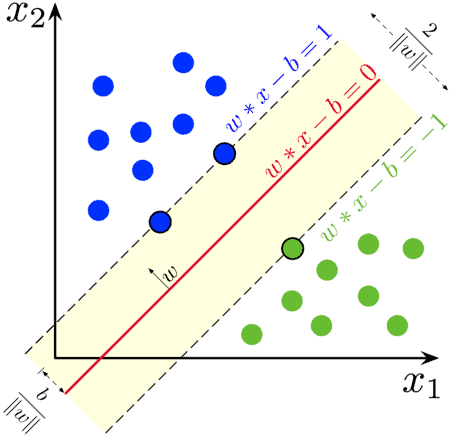## 1. Introduction

In this tutorial, we’ll be analyzing the methods Naïve Bayes (NB) and Support Vector Machine (SVM). We contrast the advantages and disadvantages of those methods for text classification. We’ll compare them from theoretical and practical perspectives. Then, we’ll propose in which cases it is better to use one or the other. During the tutorial, we’re considering the following aspects: the number of texts for training and exploitation, the length of those texts to classify, the number of categories to consider, the preprocessing process, and so on.

## 2. Naïve Bayes (NB) Classifier

Naïve Bayes (NB) allows constructing simple classifiers based on Bayes’ theorem. Thus, it assumes that any feature value is independent of the value of the other features. NB models can accomplish high levels of accuracy while estimating the class-conditional marginal densities of data.

Because of the independence assumption, NB doesn’t need to learn all possible correlations between the features. If N is the number of features, then a general algorithm requires to analyze 2N possible feature interactions, while NB only needs the order of N data points. Thus, NB classifiers can learn easier from small training data sets due to the class independence assumption. At the same time, NB is not affected by the curse of dimensionality.

The runtime complexity of the Naïve Bayes classifier is O(NK), where N is the number of features and K is the number of label classes.

## 3. Support Vector Machine (SVM)

Support Vector Machine (SVM) is a very popular model. SVM applies a geometric interpretation of the data. By default, it is a binary classifier. It maps the data points in space to maximize the distance between the two categories.
For SVM, data points are N-dimensional vectors, and the method looks for an N-1 dimensional hyperplane to separate them. This is called a linear classifier. Many hyperplanes could satisfy this condition. Thus, the best hyperplane is the one that gives the largest margin, or distance, between the two categories. Thus, it is called the maximum margin hyperplane:we can see a set of points corresponding to two categories, blue and green. The red line indicates the maximum margin hyperplane that separates both groups of points. Those points over the dashed line are called the support vectors.
Frequently happens that the sets are not linearly separable in the original space. Therefore, the original space is mapped into a higher-dimensional space where the separation could be obtained. SVMs can efficiently perform a non-linear classification using the so-called kernel trick. The kernel trick consists of using specific kernel functions, which simplify the mapping between the original space into a higher-dimensional space.

## 4. How Do Both Methods Compare?

Naïve Bayes (NB) is a very fast method. It depends on conditional probabilities, which are easy to implement and evaluate. Therefore, it does not require an iterative process. NB supports binary classification as well as multinomial one. NB assumes that features are independent between them, but this assumption does not always hold. Even though, NB gives good results when applied to short texts like tweets. For some datasets, NB may defeat other classifiers using feature selection.

SVM is more powerful to address non-linear classification tasks. SVM generalizes well in high dimensional spaces like those corresponding to texts. It is effective with more dimensions than samples. It works well when classes are well separated. SVM is a binary model in its conception, although it could be applied to classifying multiple classes with very good results.
The training cost of SVM for large datasets is a handicap. SVM takes a long time while train large datasets. It requires hyperparameter tuning which is not trivial and takes time. SVM is more attractive theoretically.

Both NB and SVM allow the choice of kernel function for each and are sensitive to parameter optimization.
Comparing the accuracy of SVM and NB in spam classification showed that the basic NB algorithm gave the best prediction results (97.8%). At the same time, SVM and NB algorithms obtained an accuracy well above 90% using parameter tuning when required.

## 5. Conclusion

In this tutorial, we analyze the advantages and disadvantages of Naïve Bayes (NB) and Support Vector Machine (SVM) classifiers applied to text classification. There is no single answer about which is the best classification method for a given dataset. Results are highly dependent on the adopted methodology, the selected features and hyperparameters, and the datasets used. Maybe, we may obtain that NB is performing better than SVM in some cases with the selected parameters. But SVM might perform better than NB with another parameter selection.